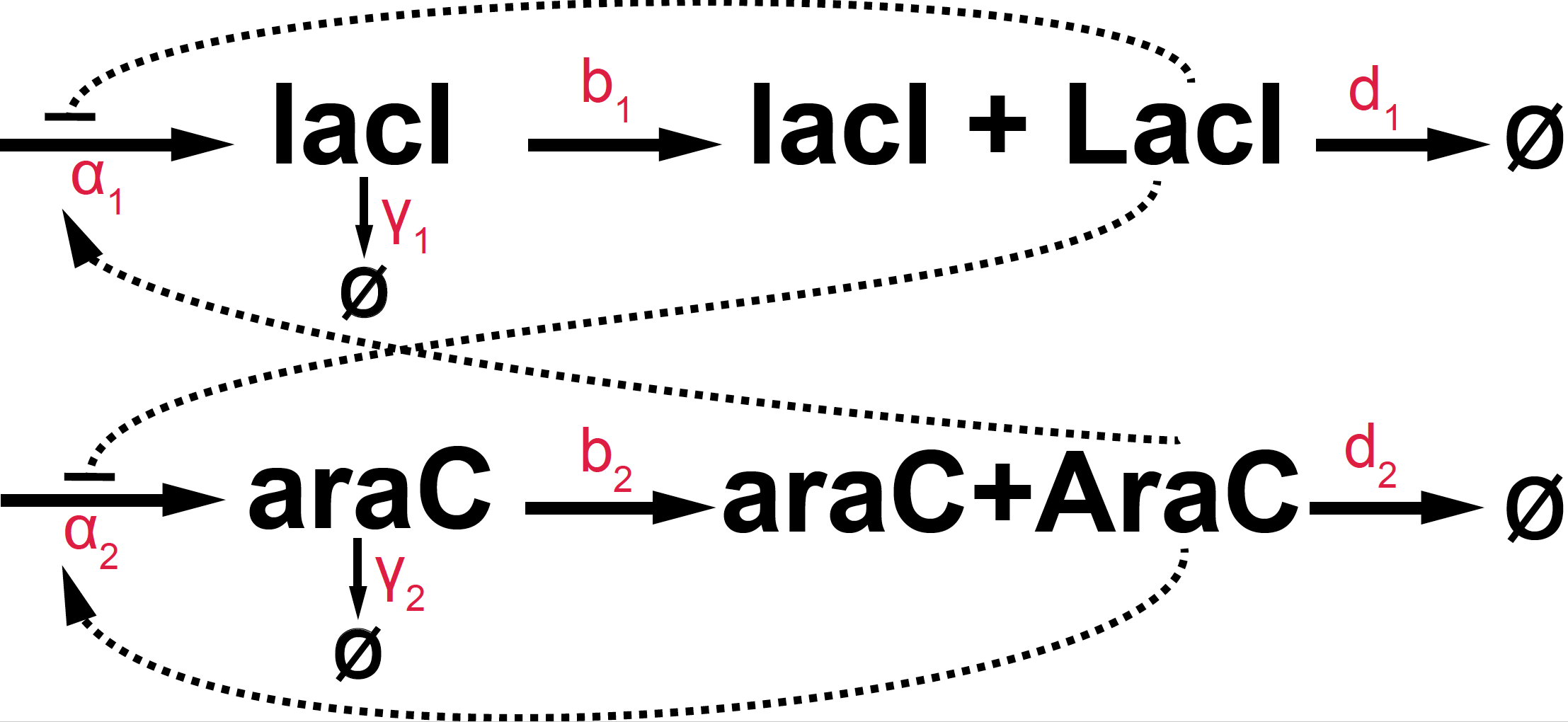# Genetic Switches

## A fast and robust oscillator

In class, we discussed the repressilator by Elowitz & Leibler, a synthetic network implemented in E. coli that produces oscillations. The repressilator turned out to be not very robust, as only 40% of the cells presented oscillations. In this homework, we are going to implement a different oscillator that depends on two genes with both negative and positive feedback loops: “A fast, robust and tunable synthetic gene oscillator” by J. Stricker et al.Figure 1. Basic diagram of the Stricker oscillator.

A diagramtic representation of this oscillator is given in Figure 1. In terms of the actual parameters:

• We know that the LacI protein acts as a dimer, and the AraC protein acts as a tetrad, which determines the Hill coefficients for the feedback loops.

• We assume enzymatic decay for all RNA and protein species with Michaelis-Menten coefficients provided bellow.

parameter Description value
$\alpha_1$ lacI RNA production 2.5
$\alpha_2$ araC RNA production 2.5
$\gamma_1$ lacI RNA degradation 0.6
$\gamma_2$ araC RNA degradation 0.8
$n1$ cooperativity coefficient of LacI binding 2
$n2$ cooperativity coefficient of AraC binding 4
$K^+$ Hill constant for LacI (-) feedback loop 2
$K^-$ Hill constant for AraC (+) feedback loop 0.003
$b_1$ lacI translation rate 0.4
$b_2$ araC translation rate 0.1
$d_1$ LacI degradation rate 0.8
$d_2$ AraC degradation rate 0.2
$K\gamma 1$ Michaelis constant for lacI RNA degradation 0.01
$K\gamma 2$ Michaelis constant for araC RNA degradation 0.1
$Kd1$ Michaelis constant for LacI degradation 0.01
$Kd2$ Michaelis constant for AraC degradation 0.1
• Propose the kinetic equations of the system.

• Solve numerically the differential equations, and plot the concentrations of the four species as a function of time, for the particular values provided in the table above.

• In your hands, is this oscillator more robust (theoretically) than the repressilator?

• Do you need the Michaelis-Menten enzymatic degradation for the system to oscillate?

• Can you identify one parameter important to maintain the oscillatory behavior? What is the range of parameters for which there are oscillations.

• Which parameter would you use to control the period of the oscillations?

• If you remove the araC gene, does the lacI system with just one negative feedback loop oscillate? How that is compare to the two-gene system of Stricker?

• The Stricker et al. implementation is slightly different from this one. You can see the details in their supplemental materials. How does your implementation compare to Stricker’s?